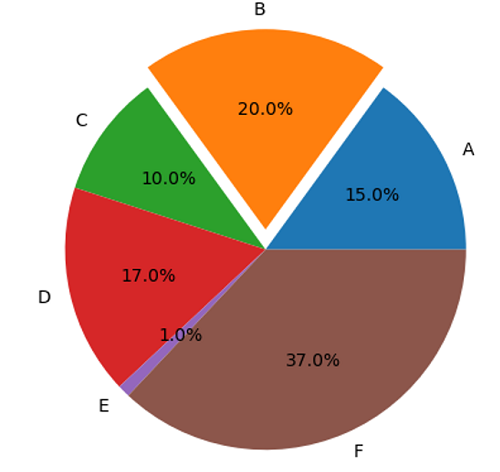# Python | Explode in Pie Plot

Explode in Pie Plot in Python. Here, we are going to learn about the Explode in Pie Plot and its Python implementation.
Submitted by Anuj Singh, on July 18, 2020

A pie plot or a pie chart is a circular statistical graphic technique, in which a circle is divided into slices with respect to numerical proportion. In a pie chart, the arc length, central angle, and area of each slice, is proportional to the quantity it represents. The sum of the total is always equal to 100 percent in the basic pie chart.

The name pie in the pie plot is derived for its resemblance to a pie that has been sliced. Pie charts are very widely used in the different types of projects and business world. Matplotlib has a defined function in matplotlib.pyplot.pie() for plotting a pie chart. In this tutorial, we are going to introduce explode functionality in pie charts by which a slice can be separated from the main pie.

The following example is an illustration of the same.

```matplotlib.pyplot.pie(
sizes,
labels=labels,
explode=explode,
autopct='%1.1f%%')
```## Python code for explode in pie plot

```# Data Visualization using Python
# Pie Chart

import matplotlib.pyplot as plt

# Pie chart, where the slices will be ordered
#  and plotted counter-clockwise:
labels = 'A', 'B', 'C', 'D', 'E', 'F'
sizes = [15, 20, 10, 17, 1, 37]
explode = (0, 0.1, 0, 0, 0, 0)

plt.figure()
plt.pie(sizes, labels=labels, explode=explode, autopct='%1.1f%%')
# Equal aspect ratio ensures that
# pie is drawn as a circle.
plt.axis('equal')

plt.show()
```

Output:

```Output is as figure
```